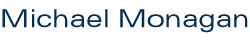[an error occurred while processing this directive]

## Topics in Computer Algebra, Summer 2011

Meet, Wednesdays, 9:30-11:30pm, K9509

### Content

• May 12. Applications of the Fast Fourier Transform:
Fast multiplication, fast division and the fast Euclidean algorithm.
• May 25. Sparse polynomial multiplication and division using geobuckets and heaps.
• June 7. Computing over Algebraic Number Fields.
- Minimal polynomials, norms, cyclotomic fields.
- The Trager-Kronecker algorithm for factoring polynomials in Q(alpha)[x].
• Sparse polynomial interpolation and computing multivariate polynomial GCDs over the integers.
- Brown's GCD algorithm using dense interpolation.
- Zippel's sparse interpolation algorithm.
- Ben-Or/Tiwari sparse interpolation.
• Introduction to computational linear algebra and rational reconstruction.
- The Bareiss fraction-free algorithm for solving Ax=b over an integral domain.
- Rational number reconstruction and solving Ax=b over Q using p-adic lifting.

### References

Algorithms for Computer Algebra by Geddes, Czapor and Labahn
Modern Computer Algebra by von zur Gathen and Gerhard
Sparse Polynomial Arithmetic by Stephen Johnson.
Polynomial division using dynamic arrays, heaps and packed exponent vectors by Monagan and Pearce.
Algebraic Factoring and Rational Function Integration by B. Trager.
A Deterministic Algorithm for Interpolating Sparse Multivariate Polynomials by M. Ben-Or and P. Tiwari.
Maximal Quotient Rational Reconstruction by Monagan.

p. (778) 782-4279 · f. (778) 782-4947 · Shrum Science K 10501 · Department of Mathematics · 8888 University Drive · Burnaby · BC · V5A 1S6 · Canada